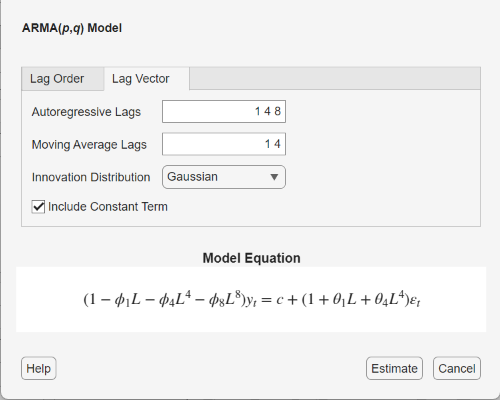ARMA Model Specifications

Default ARMA Model

This example shows how to use the shorthand arima(p,D,q) syntax to specify the default ARMA(p, q) model,

${y}_{t}=6+0.2{y}_{t-1}-0.3{y}_{t-2}+3{x}_{t}+{\epsilon }_{t}+0.1{\epsilon }_{t-1}$

By default, all parameters in the created model object have unknown values, and the innovation distribution is Gaussian with constant variance.

Specify the default ARMA(1,1) model:

Mdl = arima(1,0,1)
Mdl =
arima with properties:

Description: "ARIMA(1,0,1) Model (Gaussian Distribution)"
Distribution: Name = "Gaussian"
P: 1
D: 0
Q: 1
Constant: NaN
AR: {NaN} at lag 
SAR: {}
MA: {NaN} at lag 
SMA: {}
Seasonality: 0
Beta: [1×0]
Variance: NaN

The output shows that the created model object, Mdl, has NaN values for all model parameters: the constant term, the AR and MA coefficients, and the variance. You can modify the created model object using dot notation, or input it (along with data) to estimate.

ARMA Model with No Constant Term

This example shows how to specify an ARMA(p, q) model with constant term equal to zero. Use name-value syntax to specify a model that differs from the default model.

Specify an ARMA(2,1) model with no constant term,

${y}_{t}={\varphi }_{1}{y}_{t-1}+{\varphi }_{2}{y}_{t-2}+{\epsilon }_{t}+{\theta }_{1}{\epsilon }_{t-1},$

where the innovation distribution is Gaussian with constant variance.

Mdl = arima('ARLags',1:2,'MALags',1,'Constant',0)
Mdl =
arima with properties:

Description: "ARIMA(2,0,1) Model (Gaussian Distribution)"
Distribution: Name = "Gaussian"
P: 2
D: 0
Q: 1
Constant: 0
AR: {NaN NaN} at lags [1 2]
SAR: {}
MA: {NaN} at lag 
SMA: {}
Seasonality: 0
Beta: [1×0]
Variance: NaN

The ArLags and MaLags name-value pair arguments specify the lags corresponding to nonzero AR and MA coefficients, respectively. The property Constant in the created model object is equal to 0, as specified. The model has default values for all other properties, including NaN values as placeholders for the unknown parameters: the AR and MA coefficients, and scalar variance.

You can modify the created model using dot notation, or input it (along with data) to estimate.

ARMA Model with Known Parameter Values

This example shows how to specify an ARMA(p, q) model with known parameter values. You can use such a fully specified model as an input to simulate or forecast.

Specify the ARMA(1,1) model

${y}_{t}=0.3+0.7\varphi {y}_{t-1}+{\epsilon }_{t}+0.4{\epsilon }_{t-1},$

where the innovation distribution is Student's t with 8 degrees of freedom, and constant variance 0.15.

tdist = struct('Name','t','DoF',8);
Mdl = arima('Constant',0.3,'AR',0.7,'MA',0.4,...
'Distribution',tdist,'Variance',0.15)
Mdl =
arima with properties:

Description: "ARIMA(1,0,1) Model (t Distribution)"
Distribution: Name = "t", DoF = 8
P: 1
D: 0
Q: 1
Constant: 0.3
AR: {0.7} at lag 
SAR: {}
MA: {0.4} at lag 
SMA: {}
Seasonality: 0
Beta: [1×0]
Variance: 0.15

All parameter values are specified, that is, no object property is NaN-valued.

Specify ARMA Model Using Econometric Modeler App

In the Econometric Modeler app, you can specify the lag structure, presence of a constant, and innovation distribution of an ARMA(p,q) model by following these steps. All specified coefficients are unknown but estimable parameters.

1. At the command line, open the Econometric Modeler app.

econometricModeler

Alternatively, open the app from the apps gallery (see Econometric Modeler).

2. In the Time Series pane, select the response time series to which the model will be fit.

3. On the Econometric Modeler tab, in the Models section, click .

The ARMA Model Parameters dialog box appears.4. Specify the lag structure. To specify an ARMA(p,q) model that includes all AR lags from 1 through p and all MA lags from 1 through q, use the Lag Order tab. For the flexibility to specify the inclusion of particular lags, use the Lag Vector tab. For more details, see Specifying Lag Operator Polynomials Interactively. Regardless of the tab you use, you can verify the model form by inspecting the equation in the Model Equation section.

For example:

• To specify an ARMA(2,1) model that includes a constant, includes all AR and MA lags from 1 through their respective orders, and has a Gaussian innovation distribution:

1. Set Autoregressive Order to 2.

2. Set Moving Average Order to 1.

• To specify an ARMA(2,1) model that includes all AR and MA lags from 1 through their respective orders, has a Gaussian distribution, but does not include a constant:

1. Set Autoregressive Order to 2.

2. Set Moving Average Order to 1.

3. Clear the Include Constant Term check box.

• To specify an ARMA(2,1) model that includes all AR and MA lags from 1 through their respective orders, includes a constant term, and has t-distributed innovations:

1. Set Autoregressive Order to 2.

2. Set Moving Average Order to 1.

3. Click the button, then select t.

The degrees of freedom parameter of the t distribution is an unknown but estimable parameter.

• To specify an ARMA(8,4) model containing nonconsecutive lags

${y}_{t}-{\varphi }_{1}{y}_{t-1}-{\varphi }_{4}{y}_{t-4}-{\varphi }_{8}{y}_{t-8}={\epsilon }_{t}+{\theta }_{1}{\epsilon }_{t-1}+{\theta }_{4}{\epsilon }_{t-4},$

where εt is a series of IID Gaussian innovations:

1. Click the Lag Vector tab.

2. Set Autoregressive Lags to 1 4 8.

3. Set Moving Average Lags to 1 4.

4. Clear the Include Constant Term check box.After you specify a model, click to estimate all unknown parameters in the model.